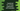# for...of loop of typescript explanation with examples# Introduction :

In this tutorial, we will learn how to use for…of loop with examples. Similar to the traditional for loop and for…in loop, we have one more variant of for loop known as the for…of loop. We can use this loop to iterate over the iterable objects like map, string, map, array etc. We will show you examples with different iterable objects. Let’s have a look :

## Syntax :

The syntax of for…of loop is as below :

``````for(let item of iterable){
//code
}``````

### for…of with an array :

We can iterate through the array elements using for…of loop like below :

``````var numArr:number[] = [1,2,3,4,5];

for(var i of numArr){
console.log(`value \${i}`);
}``````

It will print the below output :

``````value 1
value 2
value 3
value 4
value 5``````

### for…of with a string :

We can use for…of loop to iterate through the characters of a string one by one. On each iteration of the loop, it iterates through one by one character. It works only with ECMAScript 5 and above.

``````var helloStr = "Hello World!!";

for(let c of helloStr){
console.log(c);
}``````

Output :

``````H
e
l
l
o

W
o
r
l
d
!
!``````

### for…of with a map :

We can iterate through the map keys, values and entries using the for…of loop like below :

``````var map = new Map();
map.set(1,"one");
map.set(2,"two");
map.set(3,"three");
map.set(4,"four");

for(let key of map.keys()){
console.log(key);
}

for(let value of map.values()){
console.log(value);
}

for(let e of map.entries()){
console.log(e);
}``````

Note that Map is an ES6 feature. It will print the below output :

``````1
2
3
4
one
two
three
four
[ 1, 'one' ]
[ 2, 'two' ]
[ 3, 'three' ]
[ 4, 'four' ]``````

### Conclusion :

In this tutorial, we have learned how to use for…of loop in typescript with examples. It is really helpful if you don’t want the index and only the value. Try to go through the examples above and drop one comment below if you have any queries.